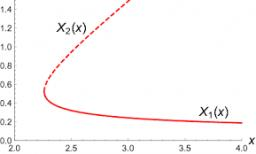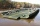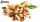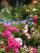# Equation with x

Solve the following equation:

2x- (8x + 1) - (x + 2) / 5 = 9

Result

x =  -1.677

#### Solution:

2x-(8x+1)-(x+2)/5=9

31x = -52

x = -5231 ≈ -1.677419

Calculated by our simple equation calculator.Our examples were largely sent or created by pupils and students themselves. Therefore, we would be pleased if you could send us any errors you found, spelling mistakes, or rephasing the example. Thank you!

Leave us a comment of this math problem and its solution (i.e. if it is still somewhat unclear...):Be the first to comment!Tips to related online calculators
Need help calculate sum, simplify or multiply fractions? Try our fraction calculator.
Do you have a linear equation or system of equations and looking for its solution? Or do you have quadratic equation?

## Next similar math problems:

1. Eq-fracSolve the following equation with fractions: h + 1/3 =5/3
2. Fraction + eqSolve following simple equation with fractions: -5/6(8+5b) = 75 + 5/3b
3. EqnSolve equation with fractions: 2x/3-50=40+x/4
4. Equation with fractionsSolve equation: ? It is equation with fractions.
5. Simple equation 6Solve equation with one variable: X/2+X/3+X/4=X+4
6. Simple equationSolve for x: 3(x + 2) = x - 18
7. EquationSolve the equation: 1/2-2/8 = 1/10; Write the result as a decimal number.
8. One thirdIf 3/5 is 360, how much is 1/3?
9. Unknown numberI think the number - its sixth is 3 smaller than its third.
10. New bridgeThanks to the new bridge, the road between A and B has been cut to one third and is now 10km long. How much did the road between A and B measure before?
11. Bag of peanutsJoe eat 1/3 of a bag of peanuts, mark eat 1/4 of the remaining in the bag of peanuts, Alvin eat 1/2 of the remaining bag of peanuts, peter eat 10 peanuts, there are 71 peanuts left. Hon many peanuts were in the bags?
12. PearsThere were pears in the basket, I took two-fifths of them, and left six in the basket. How many pears did I take?
13. FractionsThree-quarters of an unknown number are 4/5. What is 5/6 of this unknown number?
14. Cleaning windowsCleaning company has to wash all the windows of the school. The first day washes one-sixth of the windows of the school, the next day three more windows than the first day and the remaining 18 windows washes on the third day. Calculate how many windows ha
15. UN 1If we add to an unknown number his quarter, we get 210. Identify unknown number.
16. RosesOn the large rosary was a third white, half red, yellow quarter and six pink. How many roses was in the rosary?
17. Unknown numberIdentify unknown number which 1/5 is 40 greater than one tenth of that number.